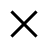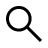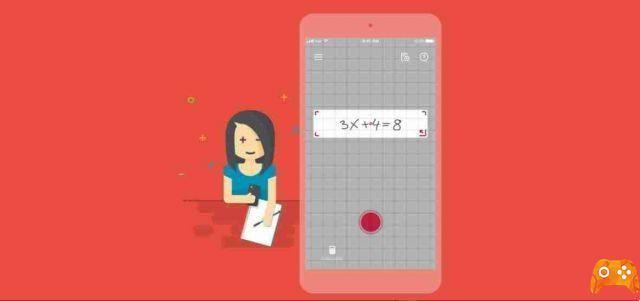Buscar

# Photomath: what it is and how it works the app that solves mathematical problemsWho I am
Martí Micolau
@martimicolauAuthor and referencesPhotomath is a mobile application described as a "calculator camera", which uses a phone's camera to recognize mathematical operations and to display the solution directly on the screen. It is available for free on Android and iOS.

## What is Photomath

Without the right support, math can get daunting. Photomath is the # 1 app for studying math that eliminates frustration and makes life as a student more peaceful.

Whether you are a math wizard or have difficulty studying it, the application will help you understand problems with comprehensive content, from arithmetic to calculations, to help you learn and understand the fundamental concepts of mathematics.

Photomath reads and solves math problems instantly, thanks to your device's camera. Check your work on printed or handwritten problems.

Learn to tackle math problems with clear and detailed instructions. For some problems you can choose between different explanation methods.

Photomath shows the calculation steps with fantastic animations, just like a professor or private teacher would do on the whiteboard.

## How Photomath works

Learn to solve math problems, check homework, and study for ACT / SAT exams and tests. More than 100 million downloads, and billions of problems solved every month! Photomath is FREE and works without wi-fi.

Instantly scan problems with printed or handwritten text using your device's camera or enter and edit equations with the scientific calculator.

Photomath breaks down each math problem into simple and easily understood steps, so that you can truly understand the fundamentals and answer questions with confidence.

## What Photomath solves

Here are some math operations that the app will help you solve:

• Basic Mathematics / Introduction to Algebra: arithmetic, whole numbers, fractions, decimal numbers, powers, roots, factors.
• Algebra: linear equations / inequalities, second degree equations, systems of equations, logarithms, functions, matrices, graphs, polynomials.
• Trigonometry / Precalculus: identity, conic sections, vectors, matrices, complex numbers, sequences and series, logarithmic functions.
• Calculus: limits, derivatives, integrals, drawing curves
Statistics: combinations, factorials.

### Related Items

##### add a comment of Photomath: what it is and how it works the app that solves mathematical problems
Comment sent successfully! We will review it in the next few hours.